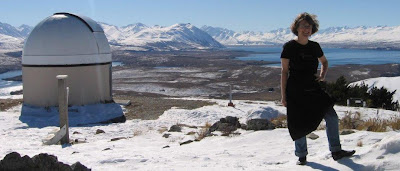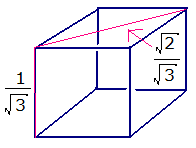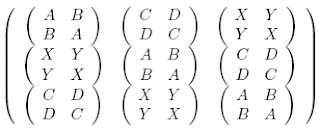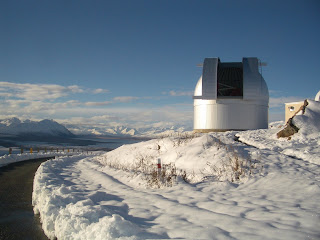occasional meanderings in physics' brave new world

## Sunday, August 31, 2008

### Mass Gap Revisited

Woit points to some new talks (not online, unfortunately) about the Mass Gap problem. Apparently Witten discussed this problem from a non-Abelian Hamiltonian point of view, and nicely avoided gauge symmetries even though the term Gauge Theory appears in the title of the talk.

Anyway, Carl has also been bugging me a bit about Hamiltonians. Honestly, I wish we could move beyond 19th century physics, but perhaps it is useful to think about what a Hamiltonian operator should be from a categorical viewpoint. To begin with, Vicary's paper is a must read, defining the harmonic oscillator entirely in categorical terms.

Let us say that a Hamiltonian is any operator sitting in an eigenvalue equation for states associated with mass-energy. Hmmmmm.

## Friday, August 29, 2008

### M Theory Lesson 219

In this old post on $E_{8}$ Carl Brannen proposes recovering the self referential nature of the algebra, or at least some deformed version of it, using a finite tower of composite states built from density operators.

Such studies use ternary (eg. qutrit) as well as qubit logic. Mixtures of qubits and qutrits occur in the Kasteleyn type recursive operators. For example, starting with $4 \times 4$ Dirac matrices inserted in a $3 \times 3$ block, one allows a deformation parameter $\frac{\pi}{12}$, a 24th root of unity. Since 24 is not a prime number, it naturally factorises into $3 \times 2^{3}$, where one factor of 2 corresponds to the doubling of roots, which also occurred in the modular relation for $B_{3}$.

Similarly, the dimension of $E_{8}$ factorises into $31 \times 2^{3}$, as noted by Kostant; see this post on the j invariant, speaking of which, as terms of a theta function, the $q$ expansion lists distances of vectors on a lattice. Amazingly, in dimension 24 there are exactly 24 nice lattices. One of these is a triple of $E_{8}$ lattices, and another example is the Leech lattice, which is secretly related to all the 24 dimensional lattices.

## Tuesday, August 26, 2008

### Strings 2008

Although we still await the upload of videos for the talks, most slides from Strings 2008 are available. The must see talks, related to twistor strings (and AdS/CFT, although we don't really care about that), include those by Dixon, Alday and Green. For blog coverage see Mottle, Jester and Woit.

Green's slides were the most surprising. I was just about to nod off after an initial inundation of stringy mumbo jumbo, when I saw the words
natural $SL(2,Z)$ generalization of Riemann zeta values.
Recall that so called zeta values are usually values of the Riemann zeta function for integer arguments. In M theory, these integers are 1-ordinals in the sense of Batanin, and are associated with 1-categorical structures. Now awake, I noticed the reference to the paper by Green et al on MZVs, which I may get around to reading eventually. The appendix is written by D. Zagier!

Update: Webcast here.## Monday, August 25, 2008

### Fardwit of the Week

From M (a regular commenter) at NEW:
I hope Rovelli knows that there is no point in insisting, as no logical argument will convince Lubos when he wants to believe something else. Debating seems as useless as rationally arguing with a female about emotionally sensible issues. (In case PC nazi-feminism really exists: this is a joke!)
Yeah, right. Really funny, dude.

Aside: Don't bother with the conversation between Mottle and Rovelli. It's disappointingly lame.

## Friday, August 22, 2008

### M Theory Lesson 218

The Secret Blogging Seminar discusses an astonishing mathematical paper on the quantum Fourier transform and geometric quantisation, the process of canonically associating a quantum system to a classical phase space.

In quantum computation, or M theory, one is used to confusing the discrete and quantum Fourier transforms, because both points and states are really just elements of a finite set and both transforms have basically the same definition. This paper focuses on the idea (due to Deligne) of a canonical (in a heavily category theoretic sense) normalisation factor for the transform. This factor is not simply $\frac{1}{\sqrt{n}}$, as commonly used by physicists concerned with unitarity, but rather a Gaussian term. For our favourite case of $n = 3$, this factor would be

$\sum_{k = 0,1,2} \frac{1}{\psi (- \frac{1}{2} k^{2})}$

where $\psi$ is the character that defines the Fourier transform. That is, it naturally involves sixth roots of unity. So the Gaussian $\frac{1}{2}$ is responsible for the introduction of twice as many roots, which occurred for instance in the modular relation associated to $B_{3}$. This normalisation is also similar to the one occurring in the choice of mass scale for Koide operators, which rely on the square roots of the masses.

## Sunday, August 17, 2008

### Neutrinos Again VIIIThe vector sum of the last post, representing neutrino mixing, traces a two step path on a cube in the group algebra space for $S_{3}$. Observe that a similar picture for the CKM matrix must be higher dimensional, since the normalisation of the diagonal (to 1) can only result in an edge of amplitude $4 \times 10^{-3}$ if many edges sum to 1. Although this magic version of the neutrino mixing matrix uses different phases from the simple Fourier product, the relation to a quantum Fourier transform is still evident.

The face diagonal may be decomposed into two steps of length $\frac{1}{\sqrt{3}}$, in the directions $(123) = \mathbf{1}$ and $(31)$. This tribimaximal path is then of the form $(XY)Z$, using the categorical convention of performing $Z$ first. Recall that the six paths of this type label the vertices of an important hexagon axiom.

## Friday, August 15, 2008

### Neutrinos Again VII

Recall that Carl's magic form for the experimentally verified tribimaximal mixing involves a sum of a 1-circulant and a 2-circulant. Such objects naturally live in a group algebra for the permutation group $S_{3}$. That is, let the 6 elements of $S_{3}$ (three 1-circulants and three 2-circulants) represent unit basis vectors for a six dimensional vector space, nominally $\mathbb{C}^{6}$. The tribimaximal mixing matrix for neutrinos is then expressed in the form

$\frac{1}{\sqrt{3}} (e^{i \theta_{1}}) + \frac{\sqrt{2}}{\sqrt{3}} (e^{i \theta_{2}})$

where $\theta_{1} = - \theta_{2} = \frac{\pi}{4}$ are phases in two complex directions, $((231),(213))$ and $((123),(321))$. Given the simplicity of the coefficients, a restriction of the number field would be feasible here. M theorists will also recognise the dimension of twistor space.

## Thursday, August 14, 2008

### Another Kea

I have been wondering about the identity of the NEW commenter, Kea2. It turns out that wikimedia has a cheesy photo:## Tuesday, August 12, 2008

### M Theory Lesson 217

As far as sets go, there are many cardinalities between $2 = | \{ 0,1 \} |$ and $\aleph_{1} = | \mathbb{C} |$. It is probably easier to start with 2 and work upwards, since Chu spaces for two truth values cover so much ground. Recall that a one dimensional truth valued matrix used the field with one element, called 1. This is combined with a zero in Set to form the truth set.

In discrete Fourier transforms, matrices have entries in the set $\{ 1, \omega, \cdots , \omega^{n-1} \} \cup \{ 0 \}$, which has $n + 1$ elements. That is, the number of truth values is the same as the number of MUB bases for dimension $n$, at least as long as $n$ is a prime power. Perhaps there is a good reason for this, especially since one of Pratt's basic examples is the $2 \times 2$ spinor. Anyway, Fourier operators with these restricted entries are a long way from requiring the whole set of complex numbers.

Returning to two point spaces: the Sierpinski space has one closed point and hence three open sets, namely the empty set, the set $\{ o \}$ and the set $\{ o , c \}$. The Chu matrix should therefore be a $2 \times 3$ matrix looking like

0 1 1
0 0 1

Now the really cool thing about the Chu calculator is that we can multiply Chu matrices together and then copy and paste the results into Blogger! Multiplying two Sierpinski spaces together we get the Chu matrix

011111
000111
001011
000001

OK, so that was multiplication. Hitting the addition button instead, we get

000111111
000000111
011011011
001001001

Multiplying the $3 \times 3$ matrix $(231)$ by itself we get

000011
001100
110000
010100
100001
001010
101000
010010
000101

Now try the Pauli swap matrix yourself. Then try a few more higher dimensional 1-circulants, and see how duality swaps them amongst themselves. What fun! And, yes, it has been snowing today.

### M Theory Lesson 216

As explained in a nice paper by Eva Schlaepfer, the Chu construction takes a suitable (symmetric monoidal closed) category $V$ containing a truth object $K$ and constructs another category from it, which has a structure ($\ast$-autonomous) closer to applications in physics. For example, the category of finite dimensional vector spaces is of this type. The objects of the new category are cospan diagrams in $V$ of the formIn the example of topological spaces, arising from the monoidal category Set, $K$ is the two point set. Usually one assumes that $V$ has pullbacks, so a cospan diagram may always be completed to form a square. If $A$ is the terminal one point set, such a pullback square would be a classifying square for the topos Set. An arrow in the new category is specified by a commuting square with target $K$ for a source span diagram $(f \otimes 1 , 1 \otimes g)$, where $(f,g): (A,B) \rightarrow (A',B')$ is a pair of arrows between the objects in $V$.

The unit for $\otimes$ in the new category is $(I,K)$, where $I$ is the unit in $V$. In other words, if finite dimensional vector spaces over $\mathbb{C}$ arose in this way, the unit one dimensional space would be specified by a pair $(\bullet,\mathbb{C})$ in Set. Observe how this resembles the quantum mechanical notion of state. From the logos point of view, thinking of $\mathbb{C}$ as a truth set which is much larger than {0,1} illustrates the logical complexity of complex spaces.

## Monday, August 11, 2008

### M Theory Lesson 215

Category theory is very good at generalising the concept of topological space. Dropping the assumption that membership of a point in an open set is a two valued entity leads to the idea of a Chu space. Vaughan Pratt runs this amazing website, complete with a Chu space calculator. A space is represented by a rectangular array with rows indexing points and columns indexing dual points, called states. The entries usually come from a simple set.

The basic two truth values {0,1} cover a lot of ground. For example, a topological space has points and open sets as dual points. The entries from {0,1} determine whether or not a point belongs to an open set. Using a table of 8 (= $2^{3}$) truth values, one can describe the category of groups. The category of all Chu spaces, with respect to a given truth value set, has an interesting self dual structure.

In M theory we also write down matrices with entries restricted to small sets. Moreover, the 0 and 1 appearing in the two dimensional spin operators do have an interpretation as Boolean truth values, since the category of finite sets is almost the same thing as a category of finite dimensional Hilbert spaces.

## Friday, August 08, 2008

### M Theory Lesson 214

The Young operator for a tableau of $n$ boxes is an element of the group algebra for the permutation group $S_{n}$. For $S_{3}$ it is described as follows. Let $R$ be the sum of all permutations which fix the rows. Similarly, $C$ is the sum of all permutations that fix the columns, with a sign for the parity of the permutation.In each case, $Y$ is the product $RC$. It will be a multiple of a primitive idempotent for the group algebra of $S_{3}$. The normalisation factors are $\sqrt{2}$ and $\sqrt{3}$, up to $i$.

### Cool Cats II

One cannot overemphasize how cool it is to think of a two point set as an example of a system of mutually unbiased bases.

Now ordinary categories are not as interesting as toposes, so we would like to extend the comparison of sets and vector spaces to generalised axioms for higher dimensional toposes. But then we had better consider multicategories as well. This is what logos theory aims to do: utilise physically important structures such as MUBs to determine a recursive series of axioms for $n$-dimensional topos like structures. Since ordinary weak $n$ categories should appear as certain algebras, this is clearly a very difficult problem. Unfortunately, working on gravity is like throwing oneself constantly against the brick wall of major problems in mathematics, so a poor physicist just has to accept this fate.

The dimension of the matrix operators that we study is the dimension $n$ of the MUB problem, which states that there are always $n + 1$ MUBs for prime power dimension. This $n$ also represents the dimensionality of the logos structure. Hence sets, or vector spaces, are two dimensional beasts, partly because the subobject classifier is specified by two elements, but more importantly because the categorical structure has two levels of arrow and the classifying square is planar.

Hence the M theorist's obsession with three object groupoids and triangles and cubes and other trinities. Most 20th century mathematics is set theoretic, meaning that it only deals with one dimensional logoses. For example, a group is usually treated as a set with extra structure (multiplication, unit, associativity). But as a category a group only has one object. It is often more natural to use groupoids, such as the groupoid of directed loops in a space, with concatenation for composition. All the loops from a given point to itself form a group anyway, so in a two point space there would be two groups sitting inside the loop groupoid.

### Cool Cats

Bob Coecke (an FQXi awardee) is one of few category theorists working close to the foundations of quantum mechanics (although the numbers are increasing at an alarming rate). Today at The Cafe he provided a link to his new paper on the categorical structure of Spekkens' toy quantum mechanics, namely $\dagger$ symmetric monoidal with basis structure (just think of pictures of little boxes and ribbons, which are easy to move around). Mutually unbiased bases are central to the discussion.

In particular, the paper discusses the category of finite sets and relations, in which the two element set acts as a system of observables, capable of modelling much of the behaviour of a qubit, just as in M theory. It will be interesting to extend this observation to a richer arithmetic setting. These days, similarities between sets and Hilbert spaces always invoke the notion of a field with one element, over which a set is just a vector space. And the natural groups to study in association with this field are the braid groups, especially at roots of unity.

Oh wait. Gee, that's what we're using to unify particle mass triplets and mixing matrices. But Dr Mottle tells us that this is completely idiotic and cannot possibly work, so maybe we should just go outside and make snowmen.

## Thursday, August 07, 2008

### More Moonshine

Tony Smith has some interesting comments on Witten's 24 dimensional manifold, naturally conjuring up Leech lattices and the like. What thoughts would a categorical M theorist have about this?

Firstly, 24 equals $3 \times 8$, which means we are really only looking at three dimensions over the octonions, and three categorical dimensions is already fairly tricky when it comes to 3-groups or groupoid structures. Higher dimensional manifolds are categorically messy objects, but simple (usually rational) surfaces arising from ribbon graphs and Fuchsian groups are more natural. Now the buckyball trinity involves surfaces of genus 0, 3 and 70. It seems a shame, therefore, to go hunting for surfaces of genus 24, when we already have surfaces of genus 3, and the 24 vertices of the permutohedron ($S_{4}$) are already lurking in the shadows.

This encoding of high classical dimensions down into surface structures (conformal field theory) is the beauty of moonshine. And since we look at the trinities as aspects of ternary logic, we expect to find not group actions, but 3-groups and groupoids. For example, the trinity $(E_8, E_7, E_6)$ (as for many group triples) can be viewed as a groupoid on three objects, each group corresponding to an object of the category.

Aside: I start my new cafe job in half an hour.

## Wednesday, August 06, 2008

### Quote of the Month

Regarding trinities, monsters and buckyball curves of genus 70, from John McKay at Lieven's blog:
Perhaps this is where to initiate a global discussion on $M$ and Witten’s 24 dim spin manifold $W$ for which he conjectures an effective $M$ action on its free loop space. I have suggested three approaches:
1. dKP (Carroll/Kodama) reduced using Norton’s replicability definition on Grunsky (=Neumann) coefficients so that the stress energy tensor = $-mn \cdot h[ \textrm{lcm}(m,n), \textrm{gcd} (m,n)]$.
2. There are 360 cusps in $MM$ (column $C$ of Conway-Norton Monstrous Moonshine). Is there an action on the cusps and elliptic marked points of all the Riemann surfaces of genus zero? A symplectic geometry? quotient?
3. Hirzebruch’s approach using Chern classes. Once Faber polynomials can be identified, we find we have replicable fns. It is then not far to $MM$ fns.
Any genuine attempts to decipher this comment are welcome.

## Tuesday, August 05, 2008

### FQXi III

FQXi rigged lottery grant winners announced here. Most of the names are well known, and not in any real need of funds. Who would have guessed. Two token women, only one of them a physicist, working on a theory that no longer agrees very well with observational data. So much for a careful analysis of the proposals.

## Monday, August 04, 2008

### M Theory Lesson 213

As well as a $2 \times 2$ Kasteleyn matrix with $3 \times 3$ circulant elements, we can also look at a $3 \times 3$ operator with two dimensional elements, of the formThis is associated to a three point Fourier transform with noncommutative coefficients described by matrices in the braid group $B_{2}$. On two strands it is only possible to create two kinds of knot: a multicrossing Hopf link, corresponding to even powers of the pseudo Pauli swap operator, or a single loop with $2n + 1$ crossings, such as the trefoil knot.

Allowing only Hopf braids, so that $B = D = Y = 0$, such a $6 \times 6$ element of $B_{6}$ looks like a diagram of three ribbon strands, because $A$, $C$ and $X$ just count powers of the $q$ factor defining the group generator. In this case, the matrix acts on a 3-vector $V$ via the ordinary Fourier transform

$V \mapsto (A \cdot 1 + C \cdot (231) + X \cdot (312)) V$

When $A = C = X = i = e^{\frac{2 \pi i}{4}}$ then $\sigma^{4} = 1$, so a full positive twist equals a full negative twist and the information of particle charge is lost. However, at a 12th root of unity a full positive twist is distinct from a full negative twist, which may be represented by the 10 crossing $\sigma^{10}$. By convention, let $\sigma^{6} = \sigma^{-6}$ determine the maximum number of crossings to be 6, that is three full twists.

## Saturday, August 02, 2008

### Moving Up IVThanks to Noriyuki San, who is working on the MOA telescope (pictured), for getting up early enough in the afternoon to catch some sunlight here at Mt John.

## Friday, August 01, 2008

### Origin of Species II

Todd Trimble kindly made the following comment:
I hope I'm not being too obnoxiously anticipatory by also remarking that the usual notion of permutative V-operad can be defined very concisely as a monoid in the monoidal category of V-species, where the monoidal product is species substitution.
This is far from being obnoxious, Todd! Struggling physicists appreciate perceptive comments from knowledgeable category theorists who can see where we are trying to go with M Theory. Indeed, although we tend to talk about operads loosely as one object multicategories, the species definition comes very close to what Rivasseau et al have in mind for redefining quantum field theory.

Note also that from the logos perspective (ie. M theoretic higher topos ideas) the category of finite sets (resp. vector spaces), as it sits in the topos Set (resp. Vect), plays an important role in attempting to define the generalised logic behind the simple operators that we associate to measurement algebras. The one major alteration to these structures that M theory requires, which comes up again and again in many guises, is the relaxation of the monoidal condition. This happens naturally with higher dimensional structures, as illustrated by Batanin's tower of coherence laws. For QFT, this forces a complete change of mathematical language, since too many concepts are only defined in categorical terms. Fortunately, everybody can understand simple diagrams of ribbons and trees.

Aside: Post written with wireless connection from my heavenly warm room amidst the fresh snow at the observatory. Can't seem to find any old skis lying about ...